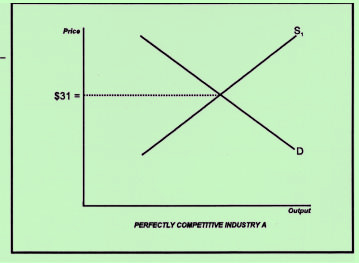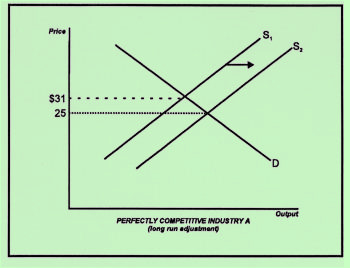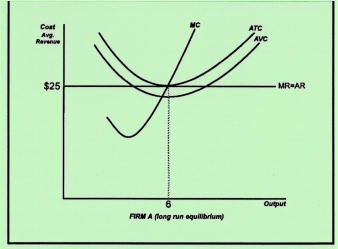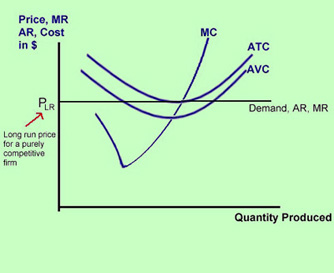Profits in Pure Competition

Because of the relative ease with which new firms can enter a purely competitive industry, it is unlikely that economic profits will be unreasonably high in the long run. In fact, economists say that for firms in perfect competition economic profits are zero in the long run. This means that there are normal or average accounting profits, but no economic profits. If in the short run economic profits are above zero (above normal accounting profits), then existing firms have an incentive to increase production. Also, venture capitalists (investors) from outside the industry will become attracted to the profit potential, and enter the industry. This increases the supply of the product and lowers the price in the market. The lower price will decrease profits until in the long run economic profits are zero again.

The reverse is also true. If firms in the industry are incurring losses, then some (the weaker ones) will go out of business. The lower supply will raise the price and increase the profits for the surviving businesses. If there is not enough demand for even one business to survive (producers of typewriters; black-and-white televisions), then the industry will cease to exist, and resources will be allocated to other, more-profitable industries (producers of personal computers; color and large-screen televisions).

Long-Run Equilibrium

Let’s analyze the long-run equilibrium for the perfectly competitive industry in the graph below. The market equilibrium price is \$31. Let’s assume that at this price firms are making positive economic (above-normal) profits.Because the profits are above normal, existing firms will increase their output, and other firms will enter this market. This shifts the market supply curve to the right (see graph below). As long as firms continue to make above-normal profits, the supply curve continues to shift to the right. Let’s assume that the supply curve shifts to S2, and that at the new equilibrium price of \$25 economic profits are zero. This is the long-run equilibrium price in this market (assuming no other changes), because there are no longer above-normal profits. Economic profits are zero, which means that each firm makes a normal (average) profit.The graphs above illustrate market demand and supply curves. The graph below illustrates a market demand and cost curves for one typical firm (firm A) in this industry. At a price of \$25, the firm is making zero economic profits. The profit maximizing quantity is 6, because this is where MC equals MR. At this quantity, the ATC curve touches the demand (AR) curve. When price (AR) and ATC are equal, the firm makes zero economic profits.Purely Competitive Firms Operate at the Lowest Point of the Average Total Cost Curve

In the graph below, the long-run price is PLR, because at this price, the firm’s average cost equals the price, and the firm makes zero economic profits. Thus, the firm is making a normal, and not an above-normal, profit. At this long-run equilibrium price, the purely competitive firm operates at the lowest point on its average total cost curve. This means that a purely competitive firm operates at maximum efficiency and charges the lowest possible price (without firms going bankrupt).Video Explanation

For a video explanation of the long run equilibrium situation for a purely competitive firm, please watch: